## Text Classification Using CNNs

In this video we introduce text classification using CNNs.

Project

Build a classifier for amazon reviews at https://github.com/lukas/ml-class/tree/master/projects/8-text-classification

## Text Classification, Embeddings and 1D Convolutions

In this tutorial we’re going to look at text classification with convolutional neural networks, using embeddings. We can apply a lot of the concepts that we introduced with image processing to text, so take a look at tutorial 3 on convolutional neural networks if you need a refresher.

## Text Processing

We have seen a few ways of processing text so far. The first one is bag of words, in which we take each word and count the number of times it occurs in a document. We end up with each word being represented by a vector, in which each vector is the size of the number of words in the document.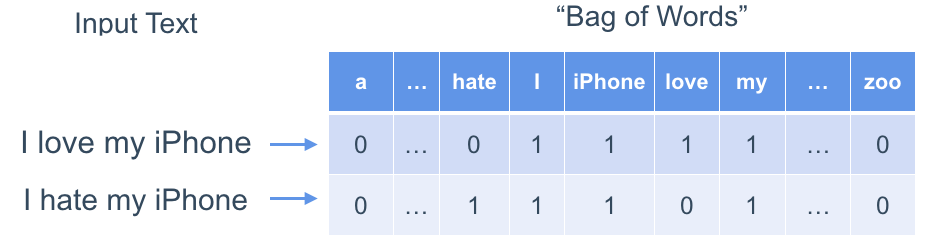The problem with bag of words however, is that you lose the order of words. The order of words matters a lot, so let’s see if there’s a better way we can do this.

The other method we have seen that takes into account the order of letters is character encoding, in which we one-hot encode every character in the text, and pad out the vectors so that all the vectors are a fixed length. The problem here however is that whole words and spaces matter a lot, and are not taken into account by character encodings.## Word Embeddings

For best results, we really want something in between character encoding and bag of words: word embeddings. We do this by taking each word and transforming it into a fixed length encoding. In this case, each word always gets mapped into the same four numbers, and so information about the word is encoded in those four values. This computation is similar to the one performed in autoencoders, in which we try and distill the meaning of a word in just a fixed length array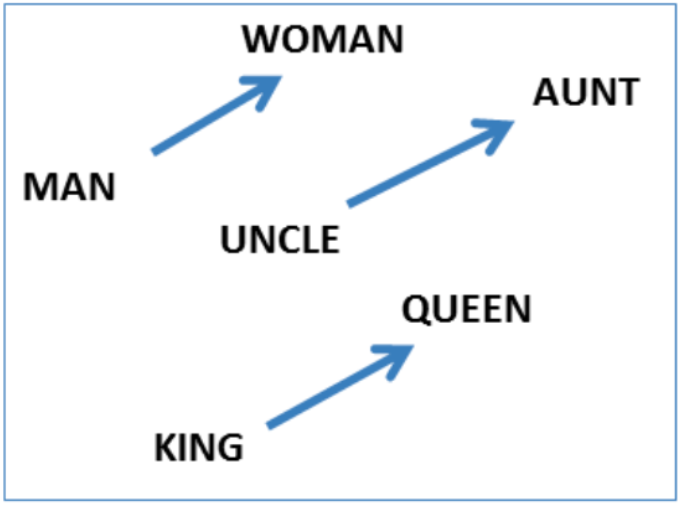We can learn these embeddings, or we can use precomputed embeddings. For instance, GloVe is word embeddings generated by Stanford that is trained on an enormous corpus of data. These embeddings have some incredibly interesting properties: for instance, if you take the embeddings for king and minus the embedding for man and finally add the embedding for woman, you actually get the embedding for queen. This shows us that the embedding is actually encoding some semantic information about the meaning of words.

We can just download these embeddings and use it on our own dataset. Now that we have these embeddings, how can we use them in our neural network?

## 2D Convolutions Review

As you may recall, convolutions help to encode relationships between neighboring pieces of data. For text, we want to encode the order of words. In order to understand 1D convolutions, it’s useful to review the 2D convolutions we did on images. We would take an input, and multiply a block of the pixel input by a some weights (kernel), and put that weighted sum in the output image. We would then keep moving the kernel along and take the weighted sum at each point, and fill in the rest of the output image.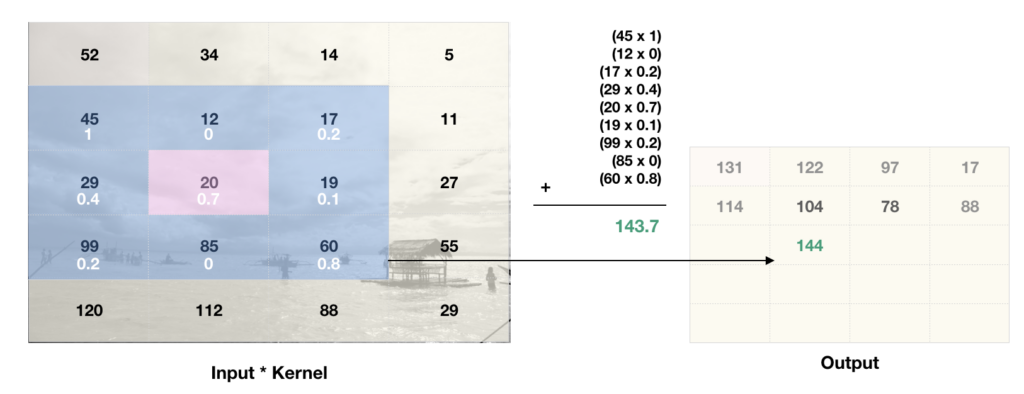We could also have multiple outputs from our convolution. To do this, we would start with the same image but we would use different sets of weights (different kernels). As we slide the block over, in each case we are multiplying the input by different values, and so output multiple images.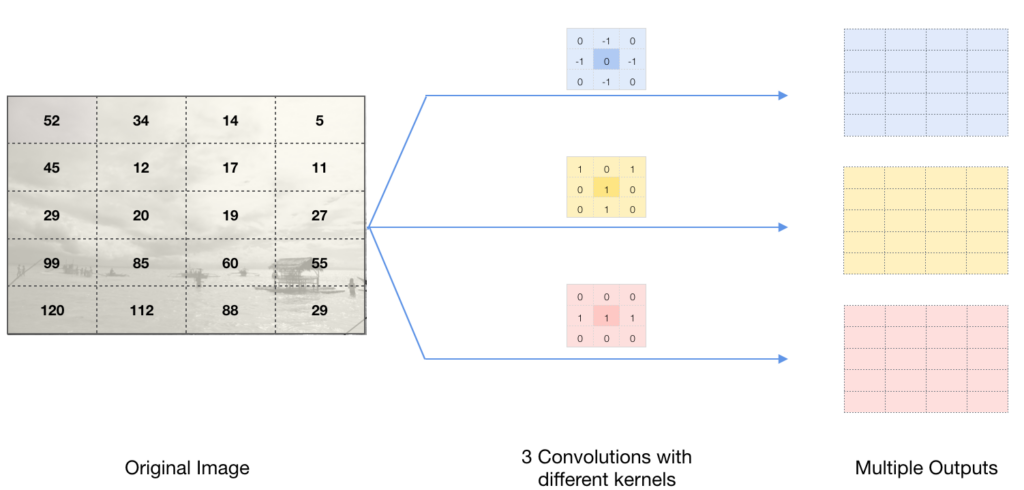We can also have multiple inputs. For instance, if we had three input images, we can use three different blocks of weights (kernels), and then sum the result of each of the blocks to produce one output.

## 1D Convolutions

For our text data, the same convolution intuition applies, however, our data is now one dimensional. We call each row in the embedding a channel. Instead of taking a 2 dimensional block, we have a 1 dimensional block (in this case length 3), across a channel. We multiply each element in the block by the weight value, and fill the weighted sum in in the output. We then run this weighted sum across all of the channels.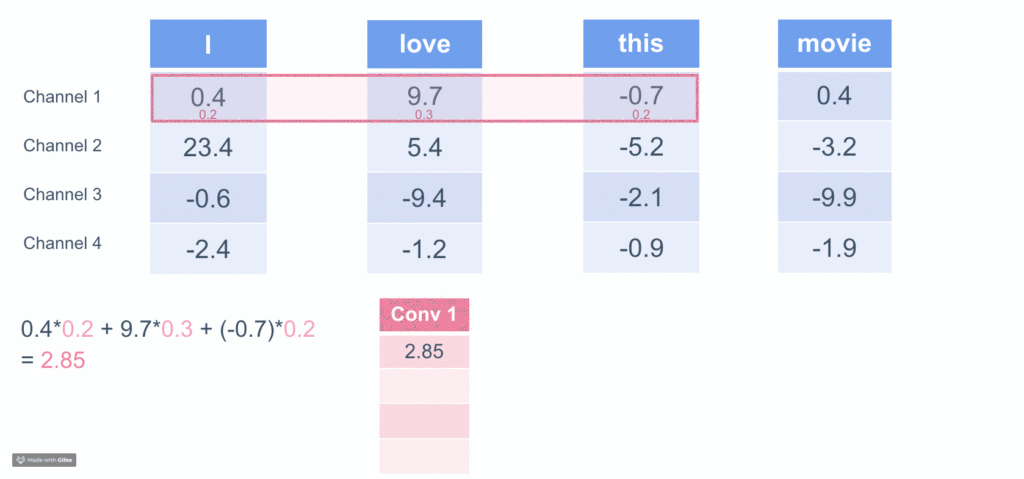We learn the weights for each of the channels. This process combines the words into smaller values – it learns information about pairs and triples of words.

## Max Pooling

You may also remember doing max pooling on images, in which we take the max value out of a block of pixels. This would shrink down the image so we could run convolutions and find patterns at multiple scales. We can also apply this operation to text. This time there is just one dimension and we do this across all channels.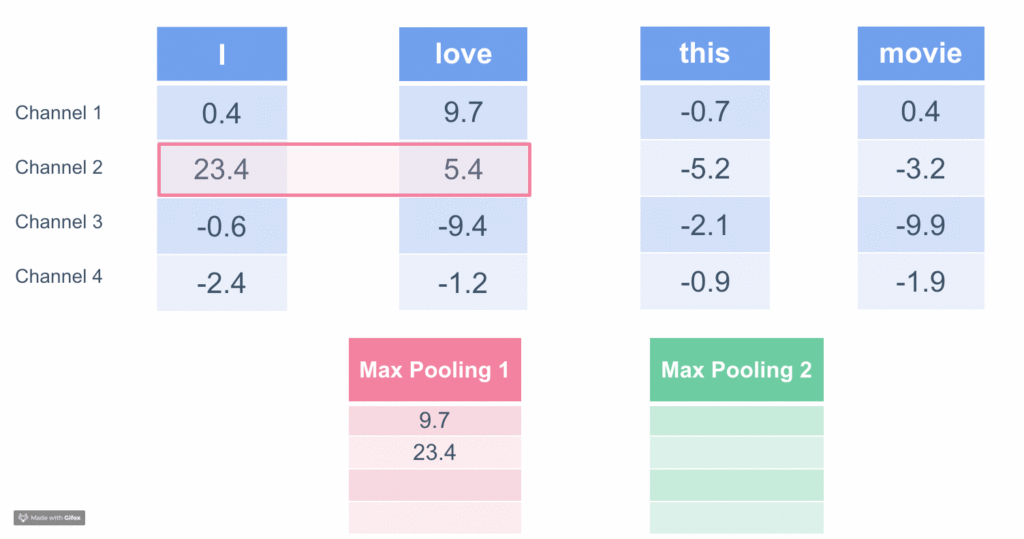It is typical to have convolutions followed by pooling followed by another convolution and so on. This helps us to find longer-range dependencies in our text. Let’s see how this translates to code.